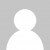JAVA爱好者

# Java精确运算（BigDecimal）

```import java.math.BigDecimal;
public class MathExtend
{
//默认除法运算精度
private static final int DEFAULT_DIV_SCALE = 10;
/**
* 提供精确的加法运算。
* @param v1
* @param v2
* @return 两个参数的和
*/
public static double add(double v1, double v2)
{
BigDecimal b1 = new BigDecimal(Double.toString(v1));
BigDecimal b2 = new BigDecimal(Double.toString(v2));
}
/**
* 提供精确的加法运算
* @param v1
* @param v2
* @return 两个参数数学加和，以字符串格式返回
*/
public static String add(String v1, String v2)
{
BigDecimal b1 = new BigDecimal(v1);
BigDecimal b2 = new BigDecimal(v2);
}
/**
* 提供精确的减法运算。
* @param v1
* @param v2
* @return 两个参数的差
*/
public static double subtract(double v1, double v2)
{
BigDecimal b1 = new BigDecimal(Double.toString(v1));
BigDecimal b2 = new BigDecimal(Double.toString(v2));
return b1.subtract(b2).doubleValue();
}
/**
* 提供精确的减法运算
* @param v1
* @param v2
* @return 两个参数数学差，以字符串格式返回
*/
public static String subtract(String v1, String v2)
{
BigDecimal b1 = new BigDecimal(v1);
BigDecimal b2 = new BigDecimal(v2);
return b1.subtract(b2).toString();
}
/**
* 提供精确的乘法运算。
* @param v1
* @param v2
* @return 两个参数的积
*/
public static double multiply(double v1, double v2)
{
BigDecimal b1 = new BigDecimal(Double.toString(v1));
BigDecimal b2 = new BigDecimal(Double.toString(v2));
return b1.multiply(b2).doubleValue();
}
/**
* 提供精确的乘法运算
* @param v1
* @param v2
* @return 两个参数的数学积，以字符串格式返回
*/
public static String multiply(String v1, String v2)
{
BigDecimal b1 = new BigDecimal(v1);
BigDecimal b2 = new BigDecimal(v2);
return b1.multiply(b2).toString();
}
/**
* 提供（相对）精确的除法运算，当发生除不尽的情况时，精确到
* 小数点以后10位，以后的数字四舍五入,舍入模式采用ROUND_HALF_EVEN
* @param v1
* @param v2
* @return 两个参数的商
*/
public static double divide(double v1, double v2)
{
return divide(v1, v2, DEFAULT_DIV_SCALE);
}
/**
* 提供（相对）精确的除法运算。当发生除不尽的情况时，由scale参数指
* 定精度，以后的数字四舍五入。舍入模式采用ROUND_HALF_EVEN
* @param v1
* @param v2
* @param scale 表示需要精确到小数点以后几位。
* @return 两个参数的商
*/
public static double divide(double v1,double v2, int scale)
{
return divide(v1, v2, scale, BigDecimal.ROUND_HALF_EVEN);
}
/**
* 提供（相对）精确的除法运算。当发生除不尽的情况时，由scale参数指
* 定精度，以后的数字四舍五入。舍入模式采用用户指定舍入模式
* @param v1
* @param v2
* @param scale 表示需要精确到小数点以后几位
* @param round_mode 表示用户指定的舍入模式
* @return 两个参数的商
*/
public static double divide(double v1,double v2,int scale, int round_mode){
if(scale < 0)
{
throw new IllegalArgumentException(“The scale must be a positive integer or zero”);
}
BigDecimal b1 = new BigDecimal(Double.toString(v1));
BigDecimal b2 = new BigDecimal(Double.toString(v2));
return b1.divide(b2, scale, round_mode).doubleValue();
}
/**
* 提供（相对）精确的除法运算，当发生除不尽的情况时，精确到
* 小数点以后10位，以后的数字四舍五入,舍入模式采用ROUND_HALF_EVEN
* @param v1
* @param v2
* @return 两个参数的商，以字符串格式返回
*/
public static String divide(String v1, String v2)
{
return divide(v1, v2, DEFAULT_DIV_SCALE);
}
/**
* 提供（相对）精确的除法运算。当发生除不尽的情况时，由scale参数指
* 定精度，以后的数字四舍五入。舍入模式采用ROUND_HALF_EVEN
* @param v1
* @param v2
* @param scale 表示需要精确到小数点以后几位
* @return 两个参数的商，以字符串格式返回
*/
public static String divide(String v1, String v2, int scale)
{
return divide(v1, v2, DEFAULT_DIV_SCALE, BigDecimal.ROUND_HALF_EVEN);
}
/**
* 提供（相对）精确的除法运算。当发生除不尽的情况时，由scale参数指
* 定精度，以后的数字四舍五入。舍入模式采用用户指定舍入模式
* @param v1
* @param v2
* @param scale 表示需要精确到小数点以后几位
* @param round_mode 表示用户指定的舍入模式
* @return 两个参数的商，以字符串格式返回
*/
public static String divide(String v1, String v2, int scale, int round_mode)
{
if(scale < 0)
{
throw new IllegalArgumentException(“The scale must be a positive integer or zero”);
}
BigDecimal b1 = new BigDecimal(v1);
BigDecimal b2 = new BigDecimal(v2);
return b1.divide(b2, scale, round_mode).toString();
}
/**
* 提供精确的小数位四舍五入处理,舍入模式采用ROUND_HALF_EVEN
* @param v 需要四舍五入的数字
* @param scale 小数点后保留几位
* @return 四舍五入后的结果
*/
public static double round(double v,int scale)
{
return round(v, scale, BigDecimal.ROUND_HALF_EVEN);
}
/**
* 提供精确的小数位四舍五入处理
* @param v 需要四舍五入的数字
* @param scale 小数点后保留几位
* @param round_mode 指定的舍入模式
* @return 四舍五入后的结果
*/
public static double round(double v, int scale, int round_mode)
{
if(scale<0)
{
throw new IllegalArgumentException(“The scale must be a positive integer or zero”);
}
BigDecimal b = new BigDecimal(Double.toString(v));
return b.setScale(scale, round_mode).doubleValue();
}
/**
* 提供精确的小数位四舍五入处理,舍入模式采用ROUND_HALF_EVEN
* @param v 需要四舍五入的数字
* @param scale 小数点后保留几位
* @return 四舍五入后的结果，以字符串格式返回
*/
public static String round(String v, int scale)
{
return round(v, scale, BigDecimal.ROUND_HALF_EVEN);
}
/**
* 提供精确的小数位四舍五入处理
* @param v 需要四舍五入的数字
* @param scale 小数点后保留几位
* @param round_mode 指定的舍入模式
* @return 四舍五入后的结果，以字符串格式返回
*/
public static String round(String v, int scale, int round_mode)
{
if(scale<0)
{
throw new IllegalArgumentException(“The scale must be a positive integer or zero”);
}
BigDecimal b = new BigDecimal(v);
return b.setScale(scale, round_mode).toString();
}
}
```

（1）、浮点数精确计算

System.out.println(0.05 + 0.01);
System.out.println(1.0 - 0.42);
System.out.println(4.015 * 100);
System.out.println(123.3 / 100);

0.060000000000000005
0.5800000000000001
401.49999999999994
1.2329999999999999

（2）、四舍五入

public double round(double value){
return Math.round( value * 100 ) / 100.0;
}

4.015 * 100 = 401.49999999999994

System.out.println(new java.text.DecimalFormat("0.00").format(4.025));
System.out.println(new java.text.DecimalFormat("0.00").format(4.0251));

4.02
4.03

（3）、浮点数输出（科学记数法）
Java浮点型数值在大于9999999.0就自动转化为科学记数法来表示，我们看下面的例子：
``` System.out.println(999999999.04); System.out.println(99999999.04); System.out.println(10000000.01); System.out.println(9999999.04); ```

9.9999999904E8
9.999999904E7
1.000000001E7
9999999.04

BigDecimal介绍

BigDecimal是Java提供的一个不变的、任意精度的有符号十进制数对象。它提供了四个构造器，有两个是用BigInteger构造，

BigDecimal(double val)
Translates a double into a BigDecimal.
BigDecimal(String val)
Translates the String representation of a BigDecimal into a BigDecimal.
BigDecimal(double)是把一个double类型十进制数构造为一个BigDecimal对象实例。
BigDecimal(String)是把一个以String表示的BigDecimal对象构造为BigDecimal对象实例。

Note: the results of this constructor can be somewhat unpredictable. One might assume that new BigDecimal(.1)
is exactly equal to .1, but it is actually equal to .10000000000000000555111512312578 27021181583404541015625.
This is so because .1 cannot be represented exactly as a double (or, for that matter, as a binary fraction of
any finite length). Thus, the long value that is being passed in to the constructor is not exactly equal to .1, appearances notwithstanding.
The (String) constructor, on the other hand, is perfectly predictable: new BigDecimal(".1") is exactly equal to .1,
as one would expect. Therefore, it is generally recommended that the (String) constructor be used in preference to this one

System.out.println(new BigDecimal(123456789.02).toString());
System.out.println(new BigDecimal("123456789.02").toString());

123456789.01999999582767486572265625
123456789.02

### 评论